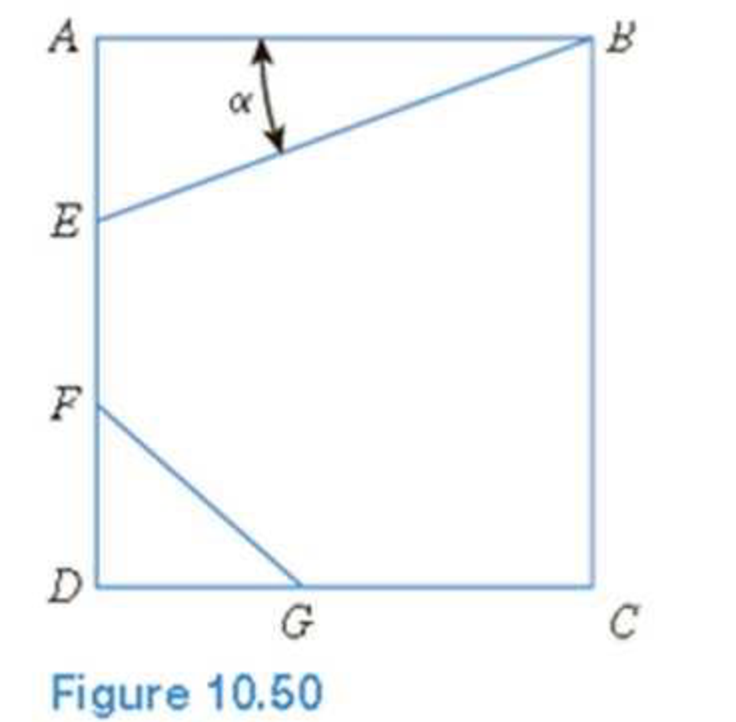Chapter 10, Problem 10.1CTPPrinciples of Geotechnical Enginee...

9th Edition
Braja M. Das + 1 other
ISBN: 9781305970939

Solutions

Chapter
SectionPrinciples of Geotechnical Enginee...

9th Edition
Braja M. Das + 1 other
ISBN: 9781305970939
Textbook Problem

EB and FG are two planes inside a soil element ABCD as shown in Figure 10.50.Stress conditions on the two planes arePlane EB: σEB = 25 kN/m2; τEB = +10 kN/m2Plane FG: σFG = 10 kN/m2; τFG = –5 kN/m2(Note: Mohr’s circle sign conventions for stresses are used above)Given α; = 25°, determine: a. The maximum and minimum principal stresses b. The angle between the planes EB and FG c. The external stresses on planes AB and BC that would cause the above internal stresses on planes EB and FG

(a)

To determine

Calculate the maximum and minimum principal stresses.

Explanation

Given information:

The stress conditions on plane AB is σEB=25kN/m2 and τEB=+10kN/m2.

The stress conditions on plane FG is σFG=10kN/m2 and τFG=5kN/m2.

The plane EB inclined at an angle (α) of 25°.

Calculation:

Apply the procedure to construct the Mohr’s circle as shown below.

• Plot the stresses in the plane EB as a point I(25,+10) and the stresses in the plane FG as a point J(10,5) on the Mohr circle

(b)

To determine

Calculate the angle between the planes EB and FG.

(c)

To determine

Calculate the external stresses in plane AB and BC that causes the internal stresses in plane EB and FG.

Still sussing out bartleby?

Check out a sample textbook solution.

See a sample solution

The Solution to Your Study Problems

Bartleby provides explanations to thousands of textbook problems written by our experts, many with advanced degrees!

Get Started

What are the benefits of daylight saving time?

Engineering Fundamentals: An Introduction to Engineering (MindTap Course List)

What test can be performed to check the efficiency of individual cylinders?

Automotive Technology: A Systems Approach (MindTap Course List)

What is a DBMS, and what are its functions?

Database Systems: Design, Implementation, & Management

Provide three examples each of closed-ended. open-ended, and range-of-response questions.

Systems Analysis and Design (Shelly Cashman Series) (MindTap Course List)